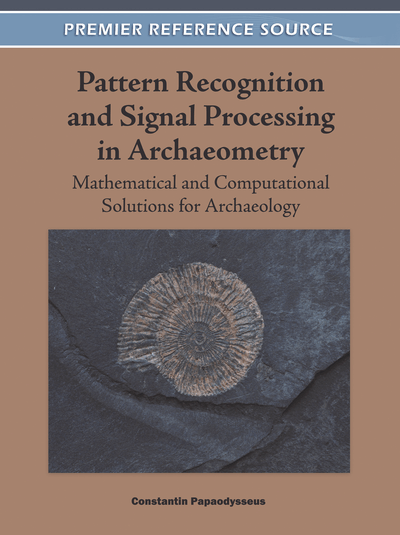# Functional Form, Elasticity and Lexical Richness: Estimates and Implications

Epaminondas E. Panas (Athens University of Economics and Business,Greece)
Copyright: © 2012 |Pages: 20
DOI: 10.4018/978-1-60960-786-9.ch005

## Abstract

One may ask whether these theoretical notions have any practical importance. For instance, does the specification of the elasticity produce appropriate indexes of lexical richness estimates? Apparently the answer is yes, as evidenced by the empirical results reported in this study.
Chapter Preview
Top

## Introduction

The index of lexical richness is a fundamental parameter describing the structure of a text. Tweedie and Baayen (1998) point out that “An obvious measure of lexical richness is the number of different words that appear in a text. Unfortunately, a text’s vocabulary size depends on its length.

This problem has led to the formulation of a number of alternative indexes of lexical richness. A good summary of much of the earlier work appears in Tweedie and Baayen (1998). There are many ways of measuring lexical richness, but the five indexes most frequently used in empirical research are Type-Token ratio, Guiraud, Brunet, Dugan and Herdan’s index.

The measures of lexical richness are based on the relationship between vocabulary (=V) and text length (=N).

Thus, the measures of lexical richness are not independent of some specification of the relationship between vocabulary (=V) and text length (=N). This is usually provided by means of a Vocabulary-Text length function or V-N function. In this case the measure of lexical richness is determined by a type functional relationship between V and N.

Two questions arise naturally. First, can such a system of indexes of lexical richness be derived from different V-N functions? And second, if the answer to the first is affirmative, what is the algebraic specification of the V-N function?

Our first objective is to develop a framework within which the V-N function can be specified. In this study, we introduce the notion of elasticity of vocabulary with respect to text length, which, in simpler terms, is the ratio of the percentage change in vocabulary to the percentage change in the text length. To determine the effects of changes in text length on vocabulary size, one needs to analyse the elasticity. Therefore, elasticity is the analytical tool that describes this impact.

Is the elasticity of vocabulary with respect to text length an appropriate consideration in the design of measures of lexical richness? To answer this question, one must understand the relationship between V-N function and elasticity.

There is no theory which would give us a basis for believing that any particular index of lexical richness is the most appropriate.

The class of V-N functions to be studied will be derived from the definition of the elasticity. We postulate that the elasticity of vocabulary with respect to text length decreases monotonically from values less than one to zero.

By specifying the elasticity, we may derive the V-N function by solving a differential Equation. The estimates of the parameters of V-N function can be used to construct measures of lexical richness. In this way indexes of lexical richness have been constructed using V-N specifications. The measure of lexical richness is incorporated in the V-N function, which is derived from the specification of the elasticity. That is, the index of lexical richness enters into the V-N function.

The use of the V-N function assures us that we can express the index of lexical richness exactly. Thus, the V-N function uniquely determines the measure of lexical richness.

Therefore, we argue that the connections between text length, vocabulary, index of lexical richness and elasticity of vocabulary with respect to text length can be expressed and can be described by what Herdan (1966) calls “the general relation between vocabulary and text length”. There is a rich literature on indexes of lexical richness. The research related to index of lexical richness includes the works of Sichel (1975, 1986) and Orlov (1983), Chitashvili and Khimaladze (1989), Good (1953) and Good and Toulmin (1956). For a comprehensive view of current applications, the reader would benefit from a recent book by Baayen (2001).

Our second objective is to exploit the V-N function in order to present empirical tests of the different measures of lexical richness, based on Ancient Greek texts.

Empirical linguistics typically provide little guidance as to the proper form of the index of lexical richness. In this study, we develop a method for testing whether a form of lexical richness is an optimal choice.

## Complete Chapter List

Search this Book:
Reset